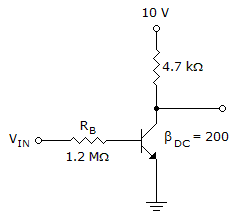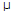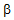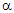# Electronic Devices - Bipolar Junction Transistors

Exercise : Bipolar Junction Transistors - General Questions
1.
Refer to this figure. Determine the minimum value of IB that will produce saturation.0.25 mA
5.325A
1.065A
10.425A
Explanation:
No answer description is available. Let's discuss.

2.
A transistor amplifier has a voltage gain of 100. If the input voltage is 75 mV, the output voltage is:
1.33 V
7.5 V
13.3 V
15 V
Explanation:
No answer description is available. Let's discuss.

3.
Refer to this figure. If VCE = 0.2 V, IC(sat) is:0.05 mA
2.085 mA
1.065 mA
7.4 mA
Explanation:
No answer description is available. Let's discuss.

4.
What is the ratio of IC to IB?DC
hFEDC
eitherDC or hFE, but notDC